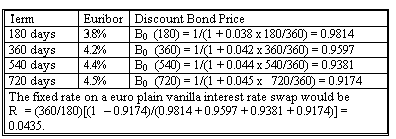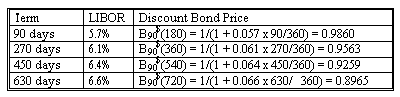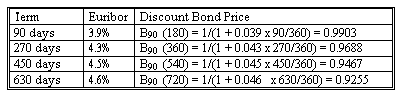### Seeing is believing!

Before you order, simply sign up for a free user account and in seconds you'll be experiencing the best in CFA exam preparation.

### Subject 4. Currency swaps

There are four types of currency swaps:

• Pay one currency fixed, receive the other fixed.
• Pay one currency fixed, receive the other floating.
• Pay one currency floating, receive the other fixed.
• Pay one currency floating, receive the other floating.

We need to determine the fixed rate, which is the rate that makes the present value of the payments made equal to the present value of the payments received.

Obviously for the last type of currency swap, in which both sides pay floating, we do not need to price the swap because both sides pay a floating rate.

Note the relationship between interest rate and currency swaps (c1: currency 1; c2: currency 2).We price currency swaps in the same way that we learned how to price interest rate swaps.

• The fixed rate on plain vanilla interest swaps in our country makes the present value of the domestic interest and principal payments equal 1.0 unit of the domestic currency.
• The fixed rate on plain vanilla interest swaps in the foreign country makes the present value of the foreign interest and principal payments equal 1.0 unit of the foreign currency.
• A notional principal of 1/S0 units of foreign currency makes the present value of the foreign interest and principal payments equal 1/S0 units of the foreign currency.
• Conversion of 1/S0 units of foreign currency at the current exchange rate of S0 gives 1.0 unit of domestic currency.
• Therefore, the present value of the domestic payments equals the present value of the foreign payments.
• The fixed rates on a currency swap are the fixed rates on plain vanilla interest rate swaps in the respective countries.

Example:

Reston Technology enters into currency swap with GSI. Reston will pay Euros at 4.35% based on NP of €10 million semi-annually for two years. GSI will pay dollars at 6.1% based on NP of \$9.804 million semi-annually for two years. Notional principals will be exchanged.

The following term structures, discount bond prices, and the resulting swap fixed rates are given below:Let dollar notional principal be NP\$. Then Euro notional principal is NP = 1/S0 for every dollar notional principal. Here Euro notional principal will be €10 million. With S0 = \$0.9804, NP\$ = \$9,804,000.

• For fixed payments, we use the fixed rate on plain vanilla swaps in that currency, R\$ or R.
• No pricing is required for the floating side of a currency swap.

During the life of the swap, we value it by finding the difference in the present values of the two streams of payments, adjusting for the notional principals, and converting to a common currency. Assume new exchange rate is \$0.9790 three months later.

Three months into the Reston-GSI swap, the new term structures and zero coupon bond prices for dollars are:The present value of the dollar fixed payments of 0.061 (180/360) plus a \$1 notional principal is: 0.061 (180/360) (0.9860 + 0.9563 + 0.9259 + 0.8965) + 1 (0.8965) = 1.01132335.

If the swap had been designed with floating payments, the present value of the dollar floating payments would be found by discounting the next floating payment, which is at the original 180-day floating rate of 5.5%, plus the market value of the floating-rate bond on the next payment day: [1 + 0.055 (180/360)] 0.9860 = 1.013115.

The new term structure and discount bond prices for the euro are:The present value of the Euro fixed payments of 0.0435 (180/360) plus a €1 notional principal is: 0.0435 (180/360) (0.9903 + 0.9688 + 0.9467 + 0.9255) + 1 (0.9255) = 1.00883078.

If the payments were floating, the present value of the euro floating payments would be found by discounting the next floating payment, which is at the original 180-day floating rate of 3.8%, plus the market value of the floating-rate bond on the next payment date: [1.0 + 0.038 (180/360)] 0.9903 = 1.0091157.

Valuation of Currency Swaps

• Dollars fixed for NP of \$9.804 million = \$9,804,000(1.01132335) = \$9,915,014.
• Dollars floating for NP of \$9.804 million = \$9,804,000(1.013115) = \$9,932,579.
• Euros fixed for NP of €10 million = €10,000,000(1.00883078) = €10,088,308.
• Euros floating for NP of €10 million = €10,000,000(1.0091157) = €10,091,157.
• Value of swap to pay € fixed, receive \$ fixed: \$9,915,014 - €10,088,308(\$0.9790/€) = \$38,560.
• Value of swap to pay € fixed, receive \$ floating: \$9,932,579 - €10,088,308(\$0.9790/€) = \$56,125.
• Value of swap to pay € floating, receive \$ fixed: \$9,915,014 - €10,091,157(\$0.9790/€) = \$35,771.
• Value of swap to pay € floating, receive \$ floating: \$9,932,579 - €10,091,157(\$0.9790/€) = \$53,336.

#### Practice Question 1

Consider a one-year currency swap with semi-annual payments. The two currencies are the US\$ and the Euro. The current exchange rate is \$0.75. The term structure of interest rates for LIBOR and Euribor are:What is the annualized fixed rate in Euros?

A. 0.0648.
B. 0.0784.
C. 0.0628.

First we compute the discount factors:
B0euro(180) = 1 / (1 + 0.06 x 180/360) = 0.9709.
B0euro(360) = 1 / (1 + 0.066 x 360/360) = 0.9381.

The fixed rate in Euro is: (1/0.5) x (1 - 0.9381) / (0.9709 + 0.9381) = 0.0648.

#### Practice Question 2

Consider a one-year currency swap with semi-annual payments. The two currencies are the US\$ and the Euro. When the two parties entered the swap, the exchange rate was \$0.75/Euro, and the term structure was as follows:The annualized fixed rates in US\$ and Euros are 7.84% and 6.48%, respectively.

Ninety days after, the term structure is as follows:If the current exchange rate is 0.7, what is the market value of the swap to pay dollar fixed and receive euro fixed?

A. -0.0712.
B. -0.0661.
C. 0.0698.

First we compute the dollar discount factors:
B90(180) = 1 / (1 + 0.071 x 90/360) = 0.9826.
B90(360) = 1 / (1 + 0.074 x 270/360) = 0.9474.

Fixed dollar payments:
The present value of the fixed dollar payments of 0.0392 (0.0784/2), including the hypothetical notional principal, is 0.0392 x (0.9826 + 0.9474) + 1 x 0.9474 = \$1.0231.

Floating dollar payments:
The 180-day dollar rate at the start of the swap was 7.2%, so the first floating dollar payment would be 0.036. The present value of the floating payments plus hypothetical notional principal of \$1 is 1.036 x 0.9826 = \$1.0180.

Then we compute the Euro discount factors:
B90euro(90) = 1 / (1 + 0.055 x 90/360) = 0.9864.
B90euro(270) = 1 / (1 + 0.06 x 270/360) = 0.9569.

Fixed Euro payments:
The present value of the fixed payments plus hypothetical 1 Euro notional principal is 0.0324 x (0.9864 + 0.9569) + 1 (0.9569) = 1.0199 euro.

Floating Euro payments:
The 180-day euro rate at the start of the swap was 6%, so the first floating euro payment would be 0.03. The present value of the floating payments plus hypothetical notional principal of 1 Euro is 1.03 x 0.9864 = 1.0160 euro.

The Euro notional principal, established at the start of the swap, is 1/0.75 = 1.3333 euro.

Now we obtain the following values for the four swaps:
• Pay dollar fixed, receive Euro fixed = -\$1.0231 + euro 1.3333 x \$0.7 x 1.0199 = -\$0.0712.
• Pay dollar fixed, receive Euro floating = -\$1.0231 + euro 1.3333 x \$0.7 x 1.0160 = -\$0.0749.
• Pay dollar floating, receive Euro fixed = -\$1.0180 + euro 1.3333 x \$0.7 x 1.0199 = -\$0.0661.
• Pay dollar floating, receive Euro floating = -\$1.0180 + Euro 1.3333 x \$0.7 x 1.0160 = -\$0.0698.